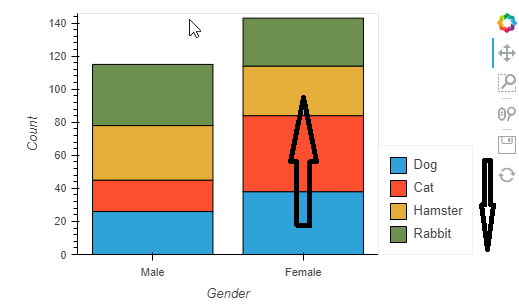# How to changed stacked Bars legend order in Holoviews

Hi

I get a stacked bar user case, And I just noticed that the order of the bar is different with the bar order.``````import numpy as np
import pandas as pd
import holoviews as hv
hv.extension('bokeh')

samples = 100

pets = ['Cat', 'Dog', 'Hamster', 'Rabbit']
genders = ['Female', 'Male']

pets_sample = np.random.choice(pets, samples)
gender_sample = np.random.choice(genders, samples)
count = np.random.randint(1, 5, size=samples)

df = pd.DataFrame({'Pets': pets_sample, 'Gender': gender_sample, 'Count': count})
bars = hv.Bars(df, kdims=['Gender','Pets']).aggregate(function=np.sum).opts(stacked=True,width = 500,legend_position='right')

bars
``````

Here is my minimized code, Is anyone know how to change the legends order align with stacked bar order?

Kind regards
Victor

I don’t think there are a way to do it right now, see this issue.

Get it Thanks for the info

Kind regards
Victor

@CongTang don’t hesitate chiming in the issue.